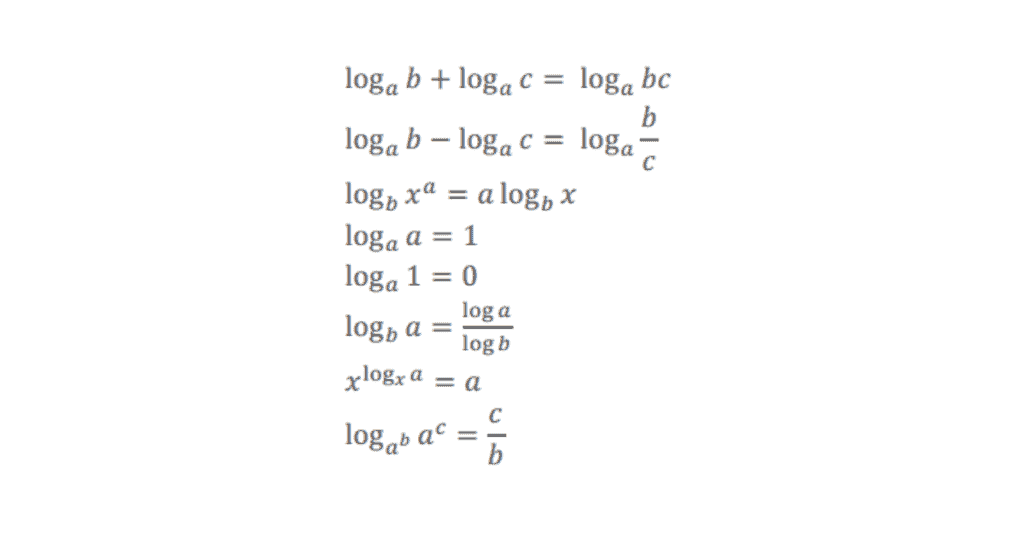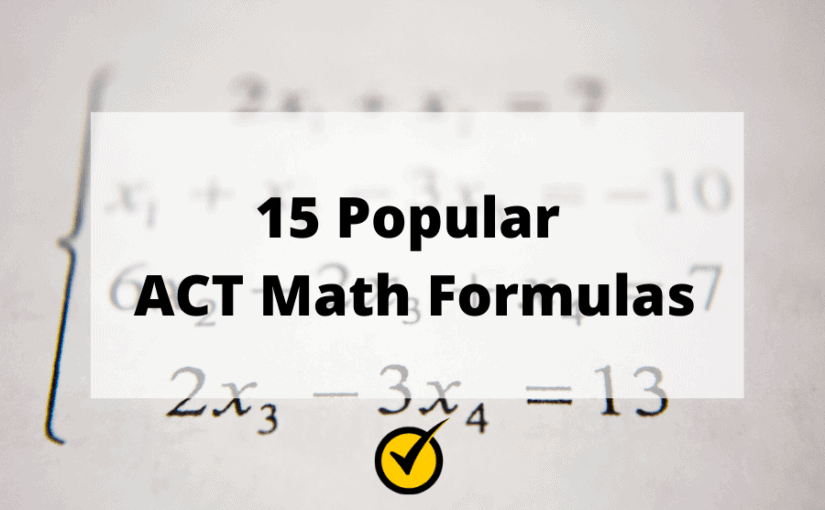# 15 Popular ACT Math Formulas

Math isn’t easy for everyone, but unfortunately, it’s unavoidable when it comes to taking the ACT exam. In fact, math makes up a large portion of the ACT exam.

While the math section may feel intimidating, there are a few things you can do to make this section a little easier for yourself.

One way to make the math section a little less intimidating is to learn what formulas you can expect to find on the ACT exam.

What is the ACT Test? What You Need to Know

However, the thing about the ACT is that you won’t be given a cheat sheet with all of the math formulas that you’ll need to know. It’s up to you to memorize them.

So to help you with that, we put together 15 of the most popular ACT math formulas that you can expect to find on the exam.

## What to Expect on the ACT Math

There are 60 multiple-choice questions from six areas of math:

• Pre-Algebra / Elementary Algebra: 24 questions
• Intermediate Algebra / Coordinate Geometry: 18 questions
• Plane Geometry / Trigonometry: 18 questions

ACT Test Prep Course

## 15 Popular ACT Math Formulas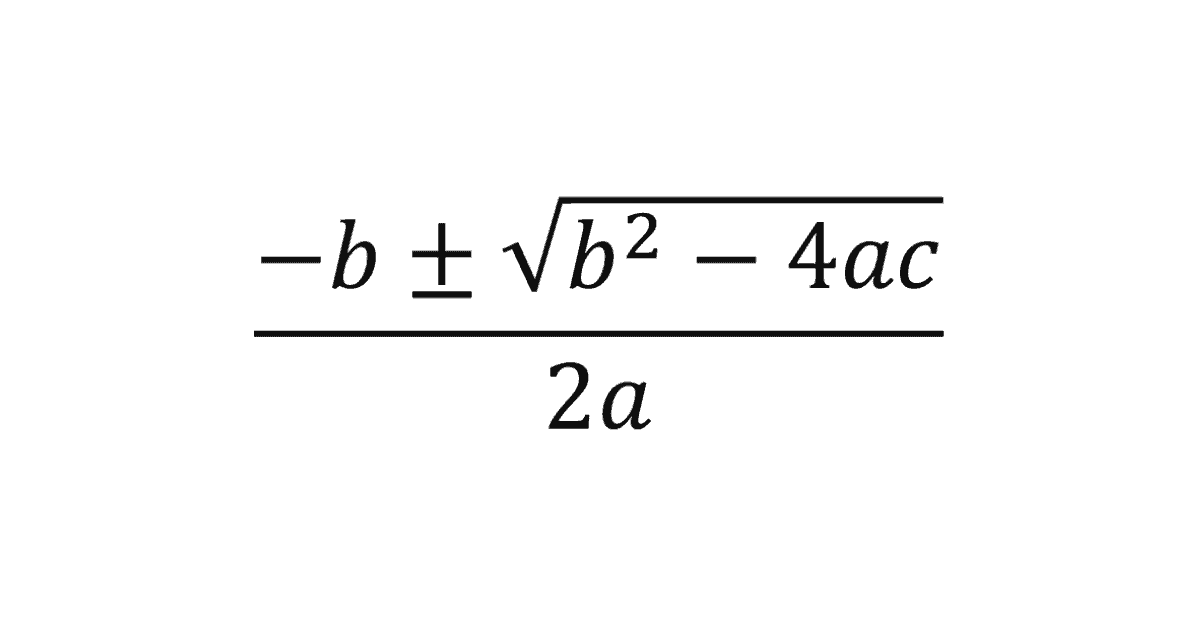2. Area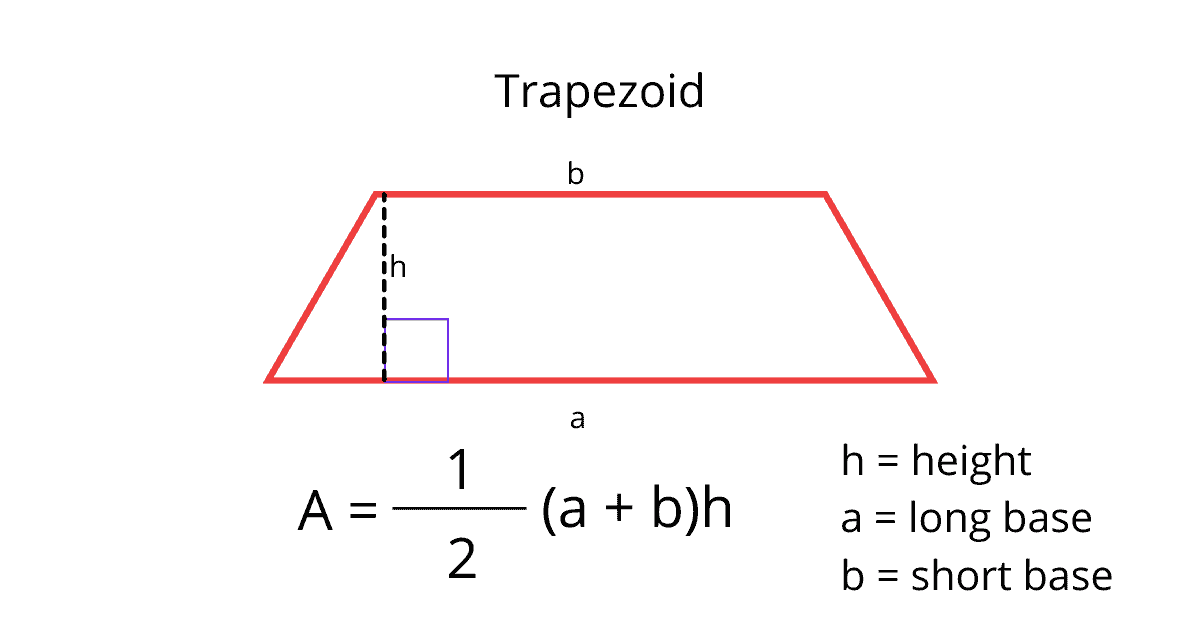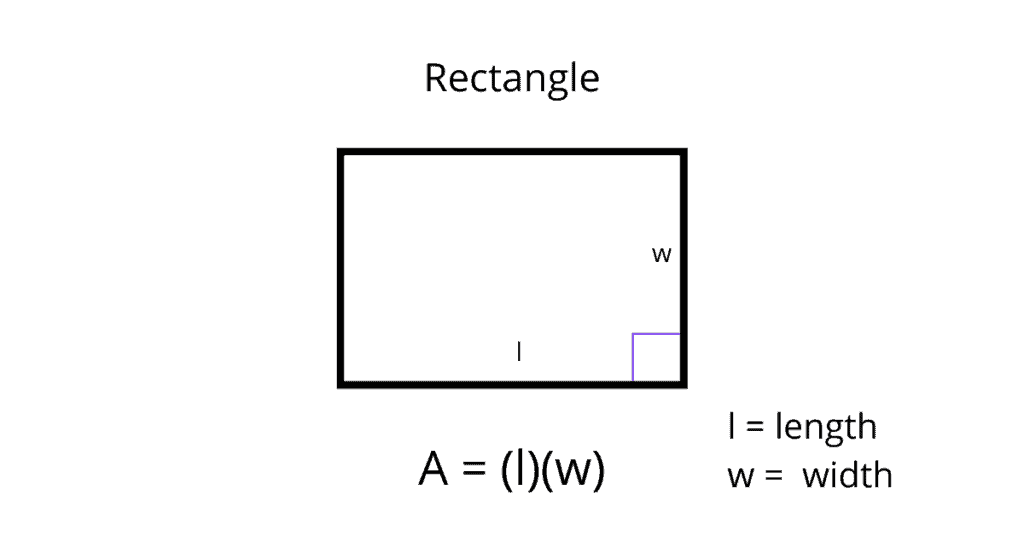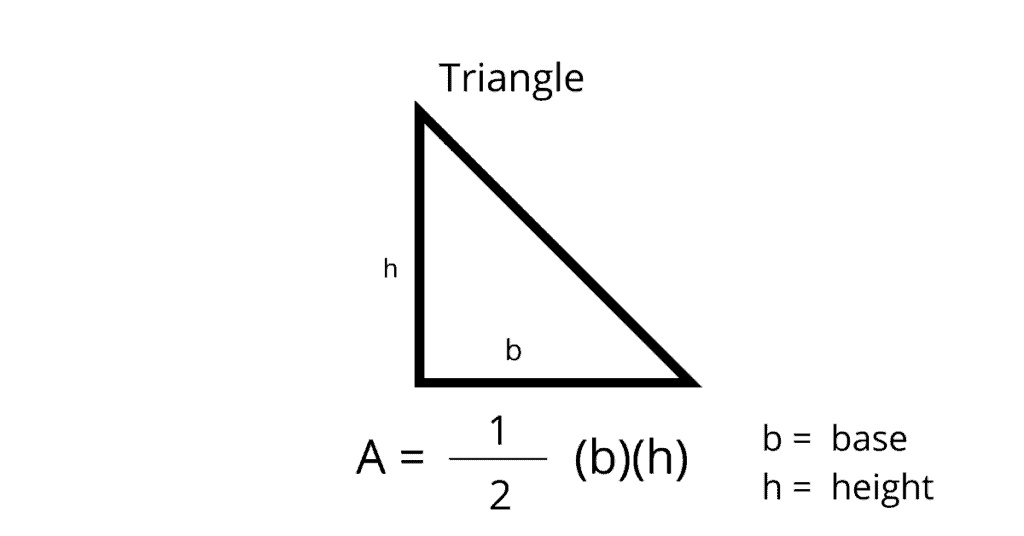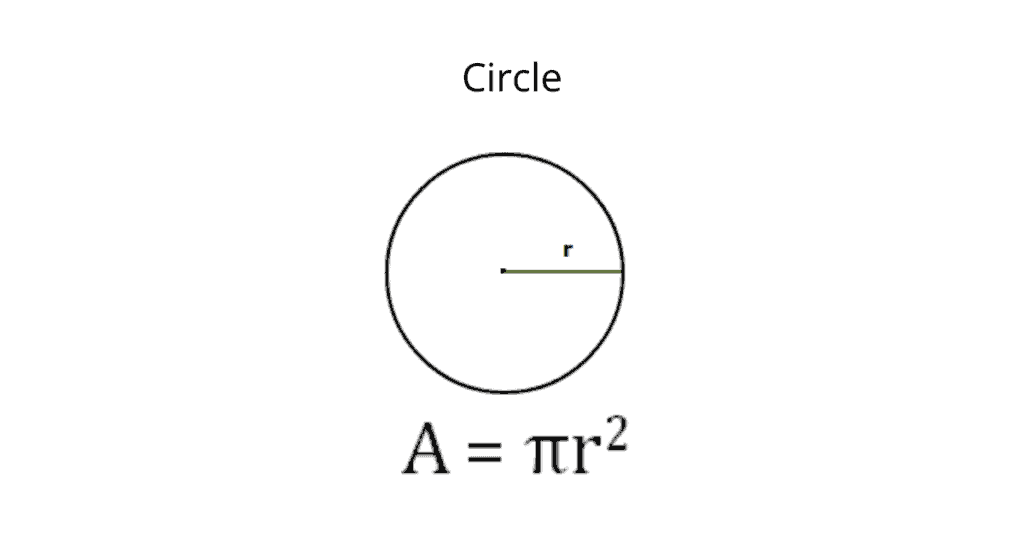3. Volume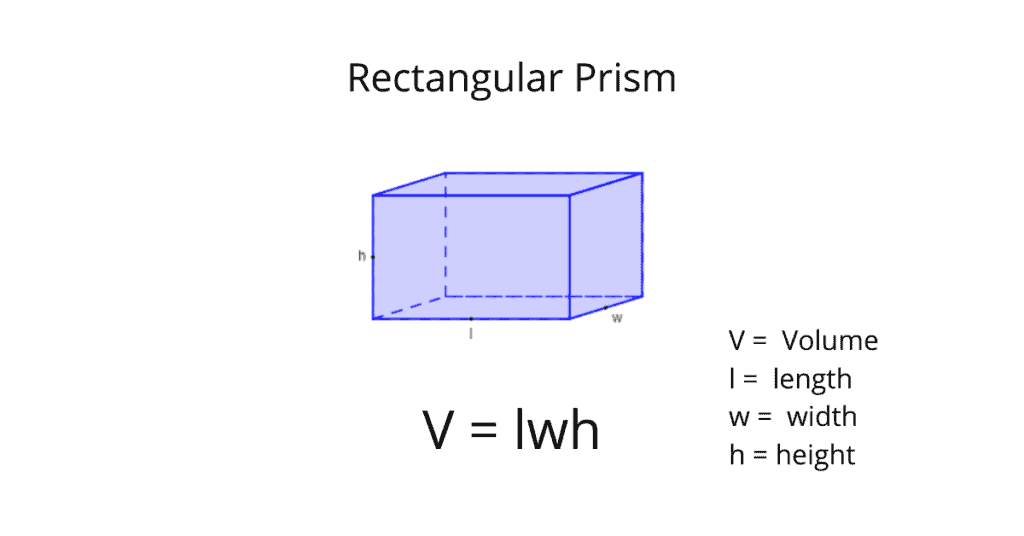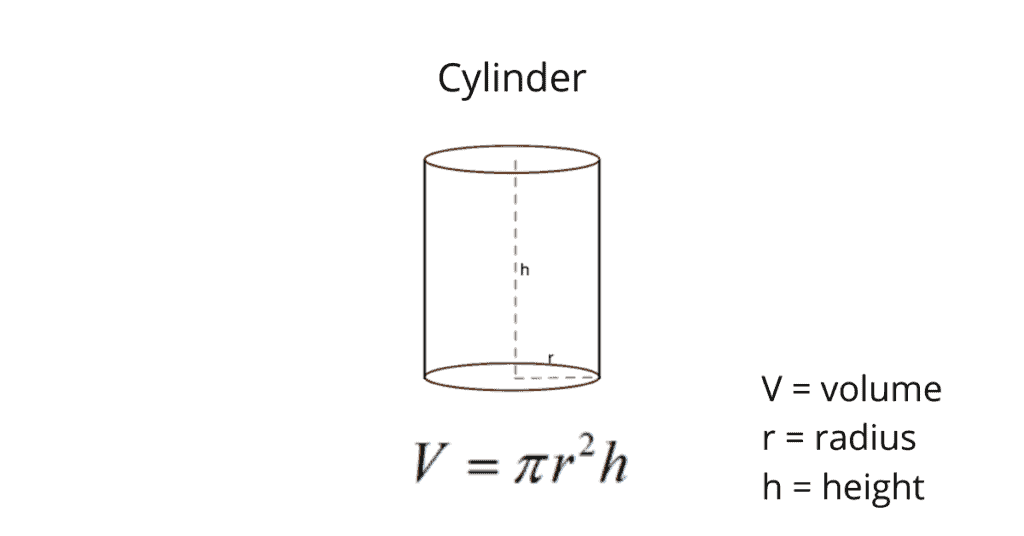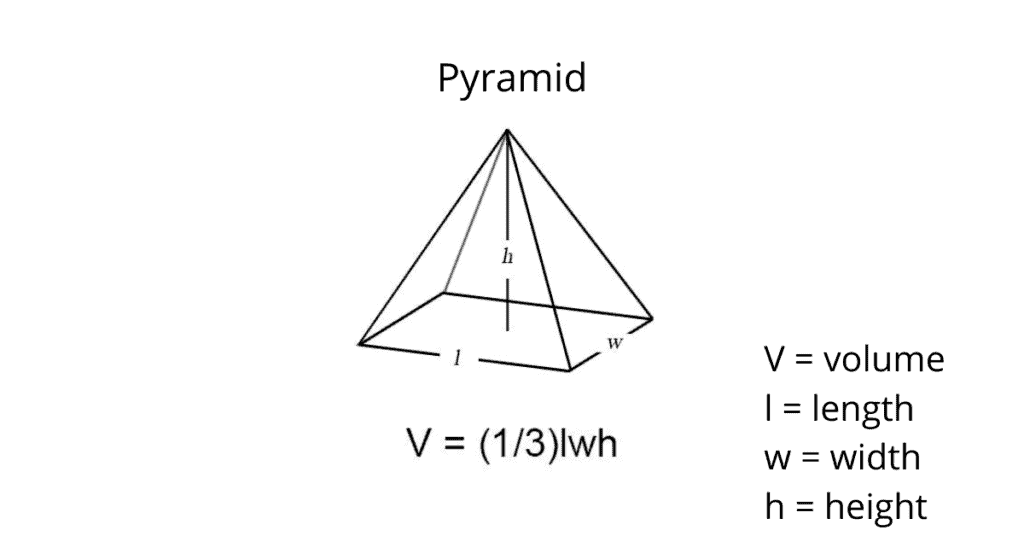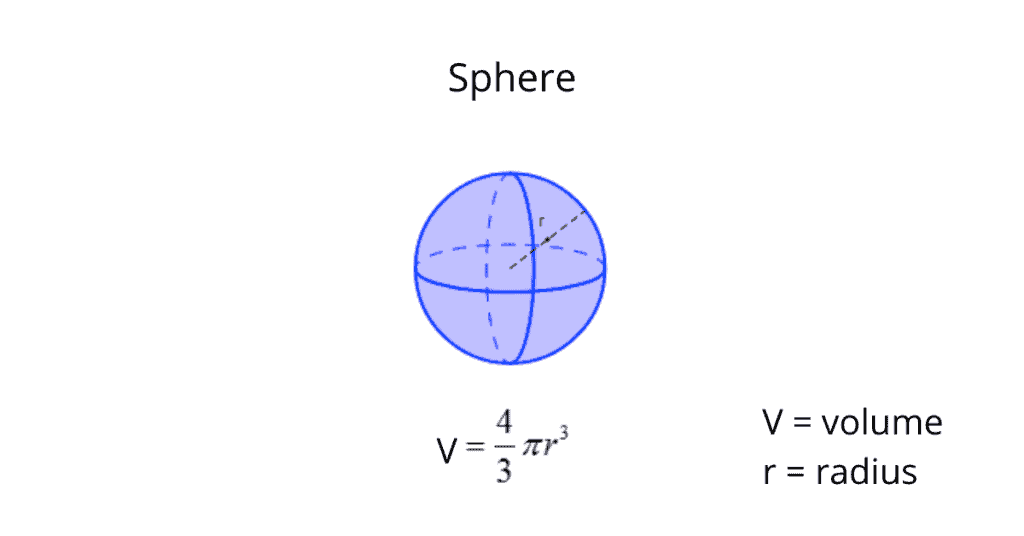4. Surface Area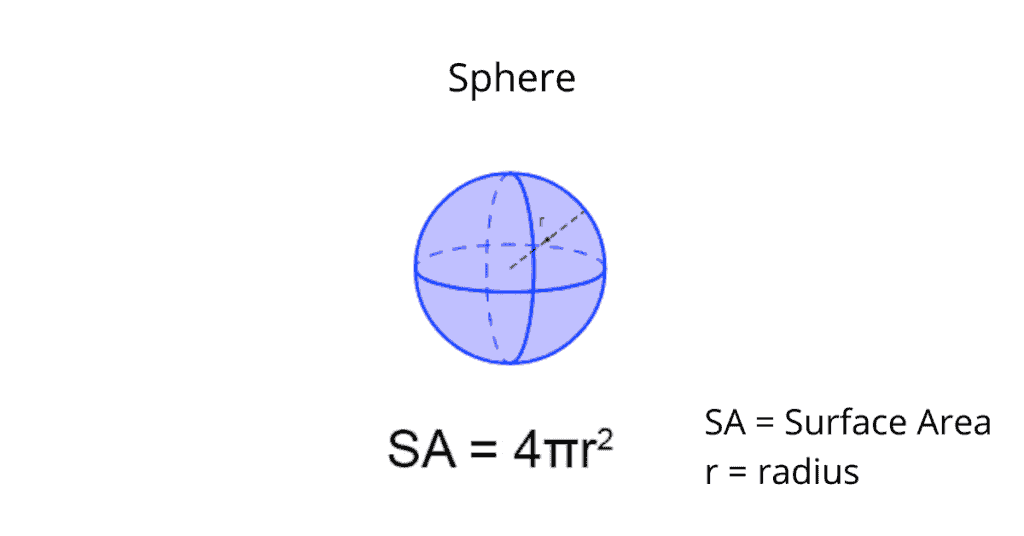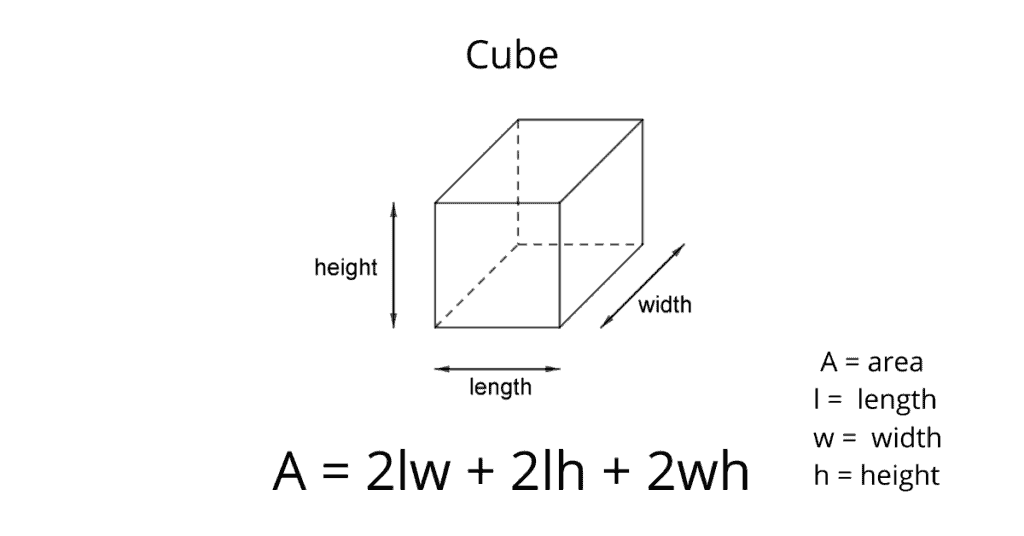5. Perimeter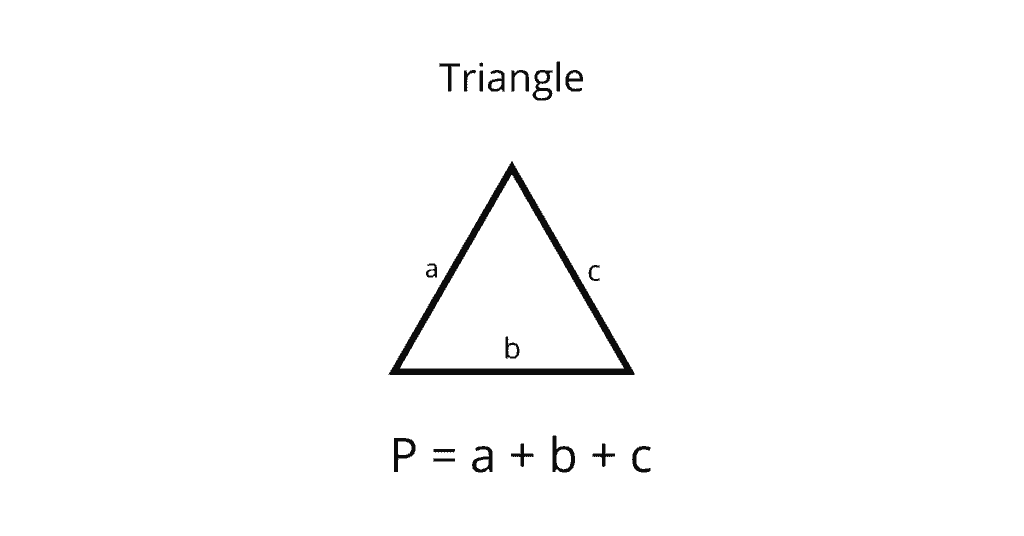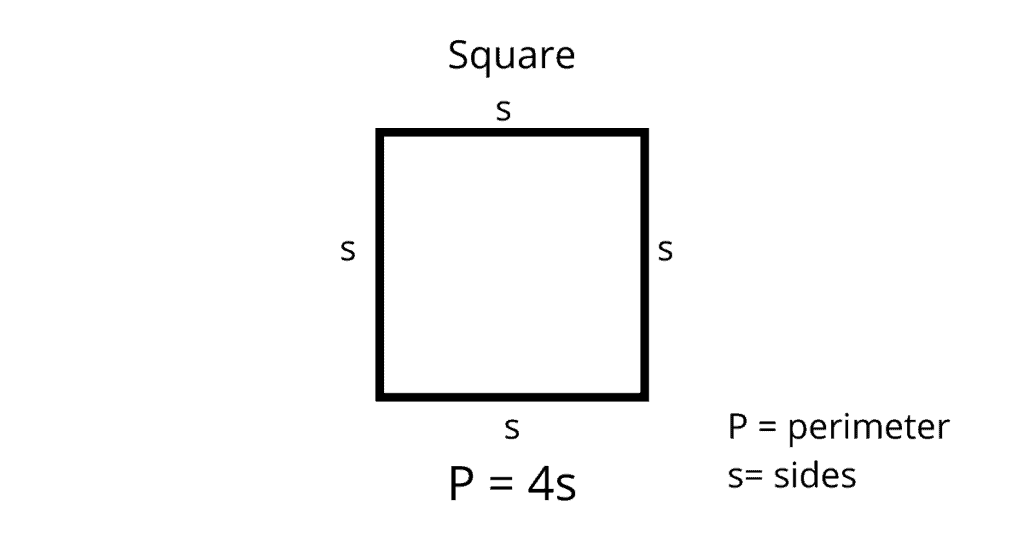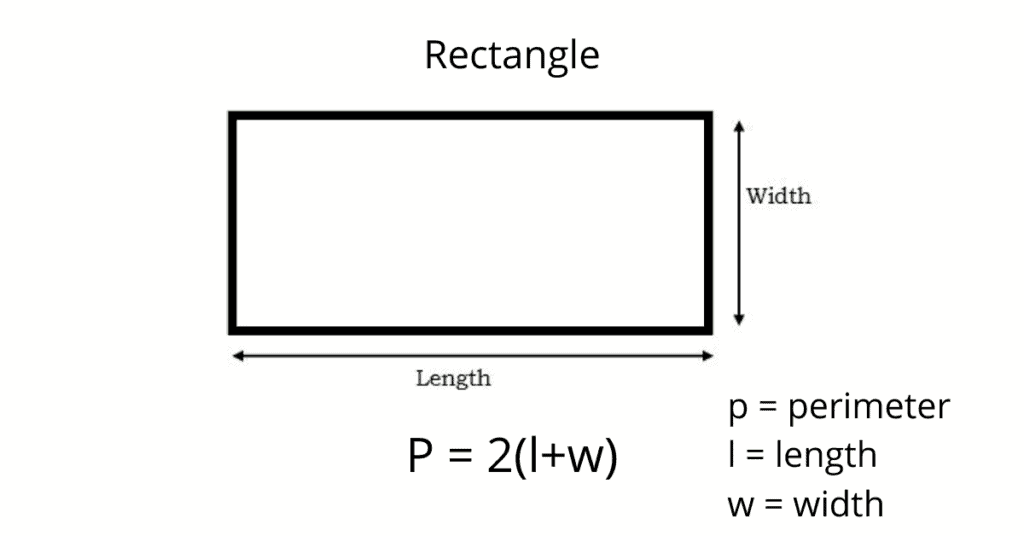6. Averages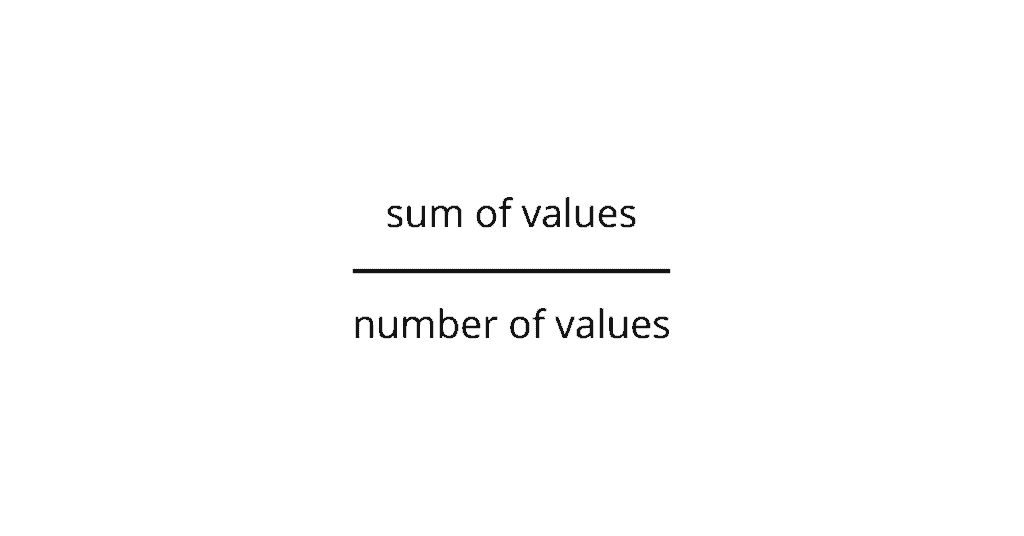7. Slope-Intercept Form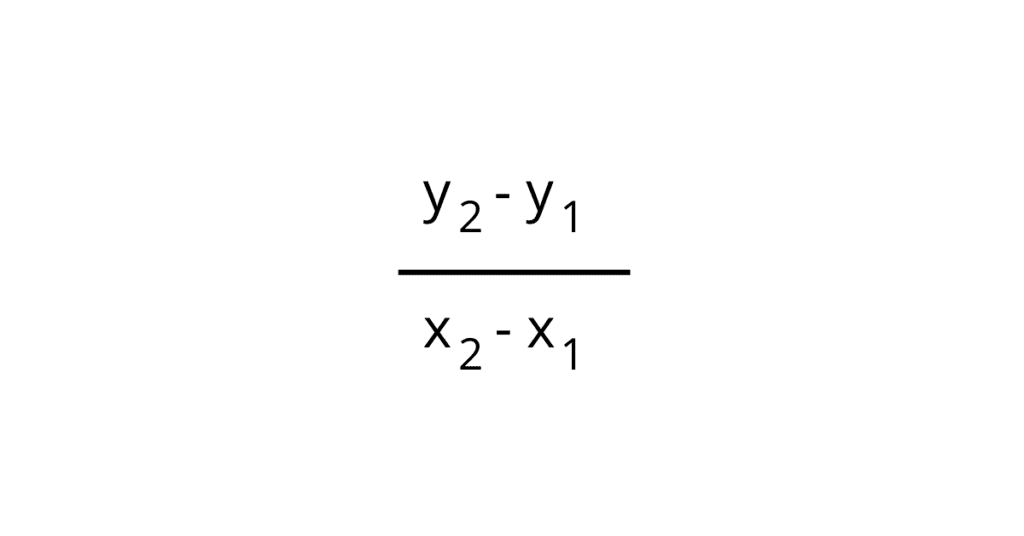8. Pythagorean Theorem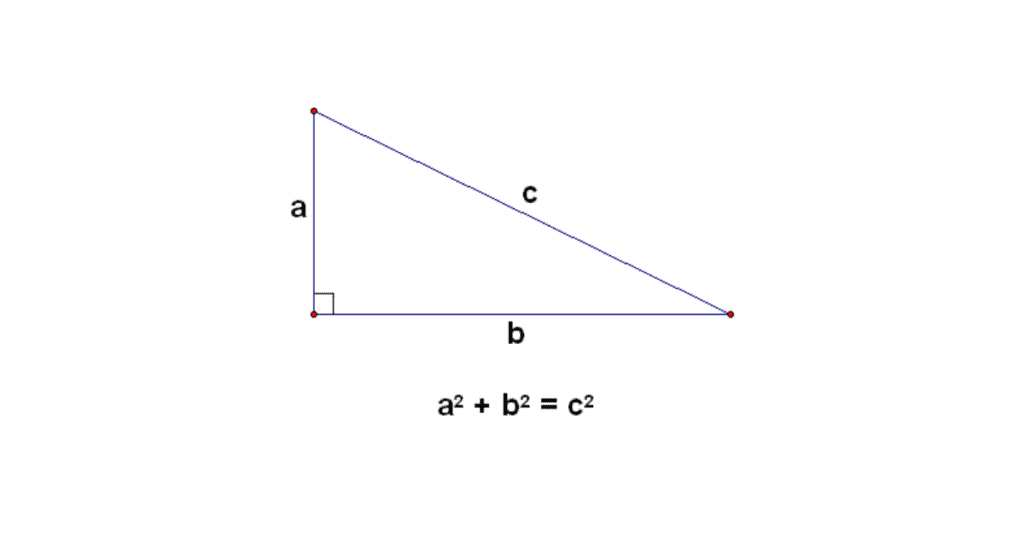9. Exponents and Roots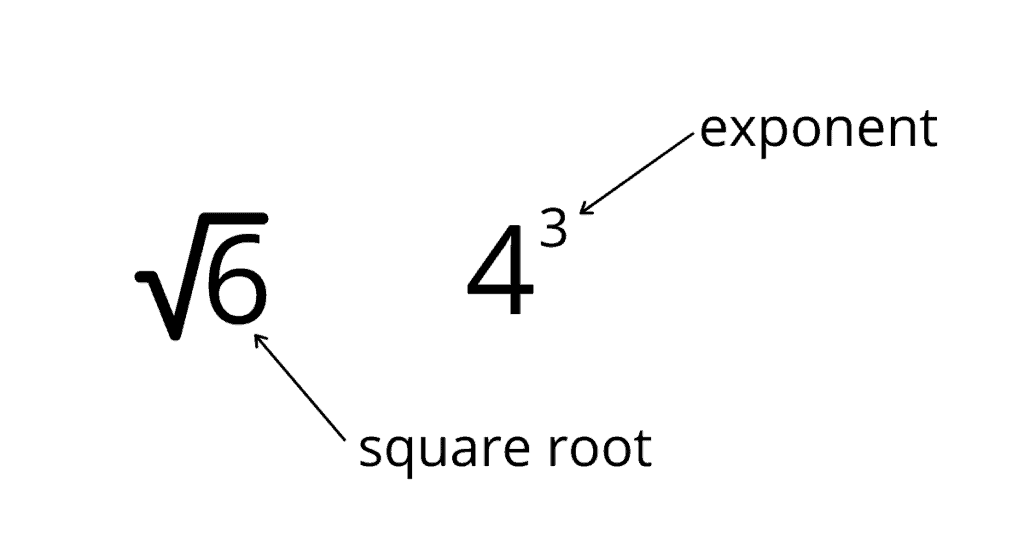10. Non-Right Triangles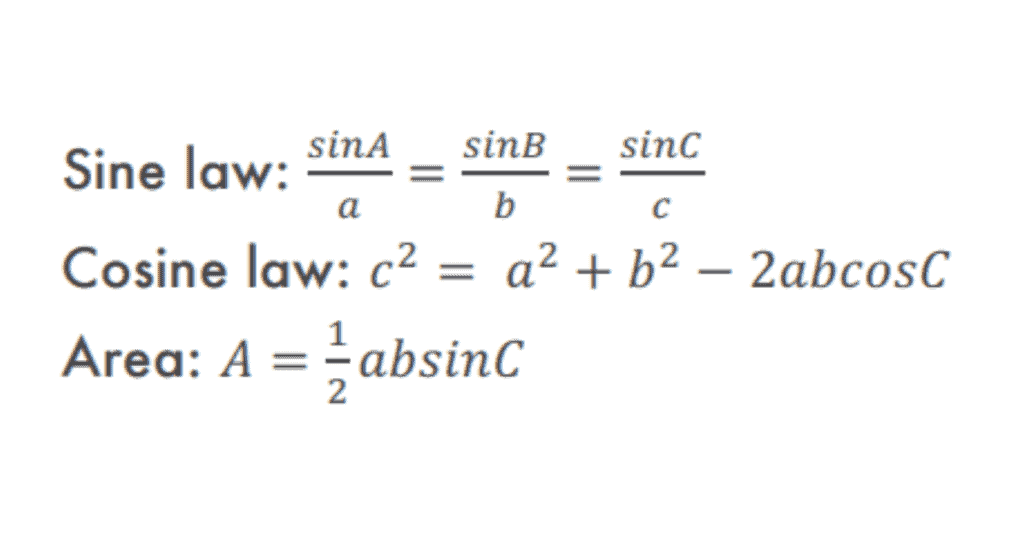11. Trigonometric Identities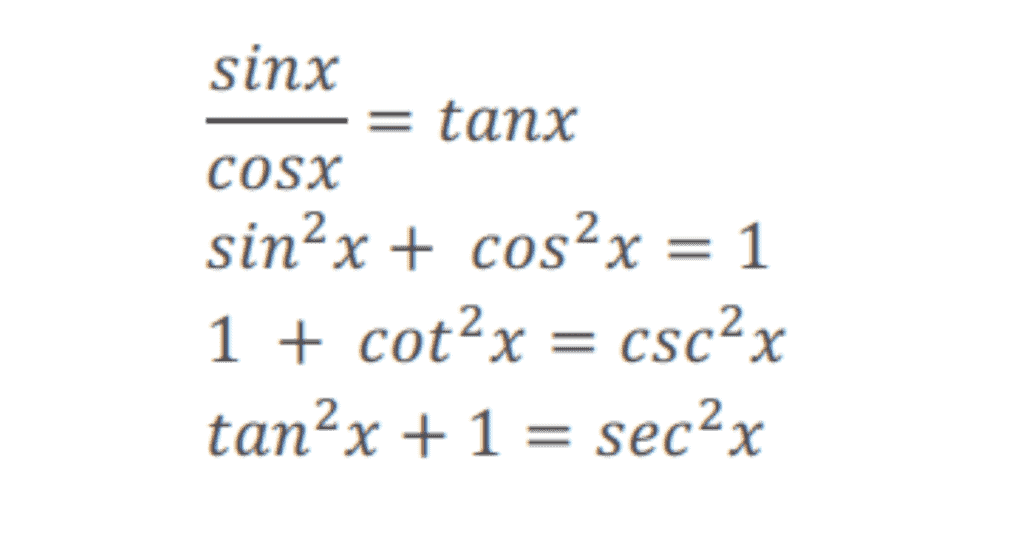12. Probability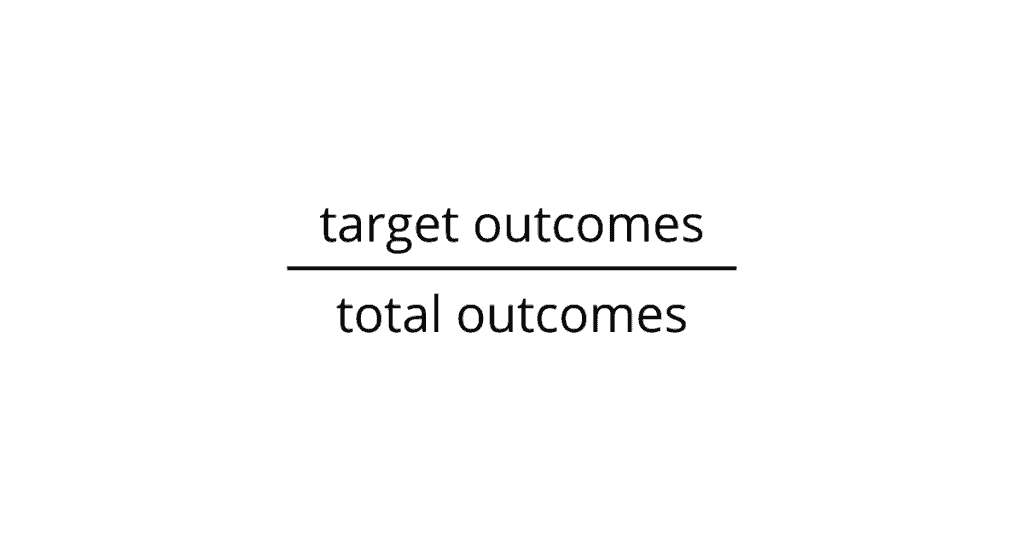13. Distance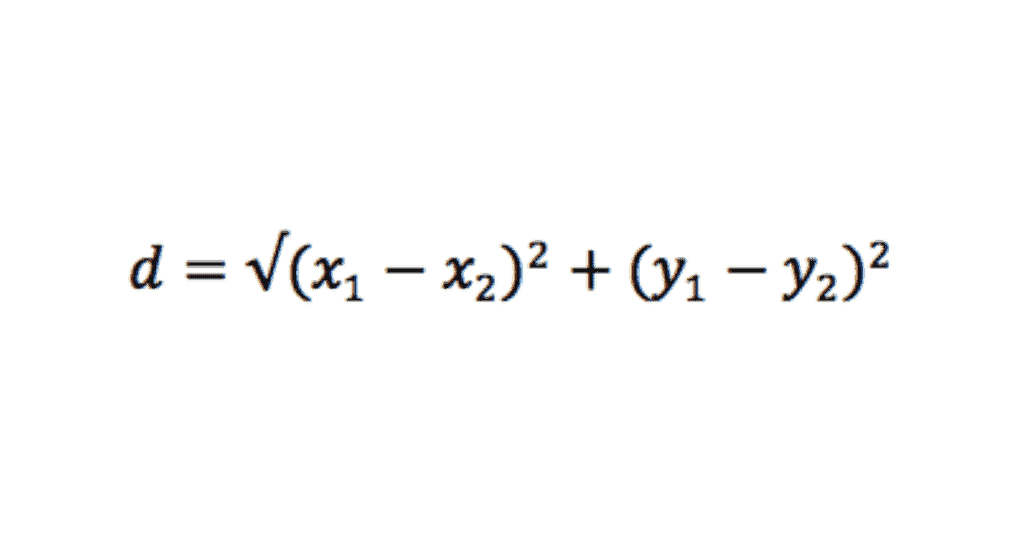14. Midpoint Formula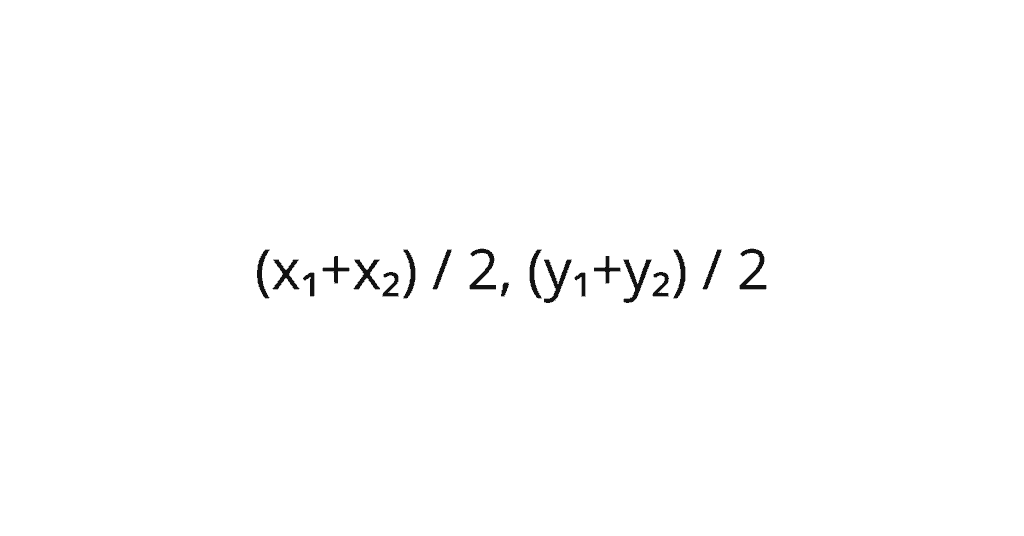15. Log